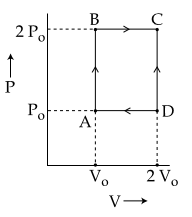#### An engine operates by taking n moles of an ideal gas through the cycle ABCDA shown in figure.  The thermal efficiency of the engine is :(Take Cv=1.5 R, where R is gas constant)Option 1)  0.24 Option 2) 0.15 Option 3)  0.32   Option 4)  0.08As we learnt in

Specific heat of gas at constant volume -- wherein

f = degree of freedom

R= Universal gas constant

Thermal Efficiency of CycleGross Heat absorved is amount of heat that is absorbed only.These two are not the part of gross heat.For monoatomic gas f = 3Correct option 2

Option 1)

0.24

This is an incorrect option.

Option 2)

0.15

This is the correct option.

Option 3)

0.32

This is an incorrect option.

Option 4)

0.08

This is an incorrect option.

#### perimeter